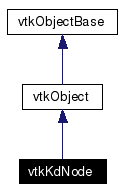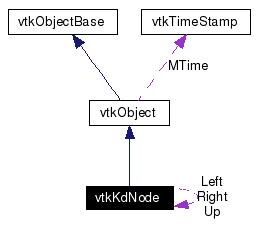# vtkKdNode Class Reference

`#include <vtkKdNode.h>`

Inheritance diagram for vtkKdNode:[legend]
Collaboration diagram for vtkKdNode:[legend]
List of all members.

## Detailed Description

This class represents a single spatial region in an 3D axis aligned binary spatial partitioning. It is assumed the region bounds some set of points. Regions are represented as nodes in a binary tree.

vtkKdTree vtkOBSPCuts

Definition at line 40 of file vtkKdNode.h.

## Public Types

typedef vtkObject Superclass

## Public Member Functions

virtual const char * GetClassName ()
virtual int IsA (const char *type)
void PrintSelf (ostream &os, vtkIndent indent)
virtual void SetDim (int)
virtual int GetDim ()
virtual void SetNumberOfPoints (int)
virtual int GetNumberOfPoints ()
void SetBounds (double x1, double x2, double y1, double y2, double z1, double z2)
void GetBounds (double *b) const
void SetDataBounds (double x1, double x2, double y1, double y2, double z1, double z2)
void GetDataBounds (double *b) const
void SetDataBounds (float *v)
double * GetMinBounds ()
double * GetMaxBounds ()
void SetMinBounds (double *mb)
void SetMaxBounds (double *mb)
double * GetMinDataBounds ()
double * GetMaxDataBounds ()
void SetMinDataBounds (double *mb)
void SetMaxDataBounds (double *mb)
virtual void SetID (int)
virtual int GetID ()
virtual int GetMinID ()
virtual int GetMaxID ()
virtual void SetMinID (int)
virtual void SetMaxID (int)
void AddChildNodes (vtkKdNode *left, vtkKdNode *right)
void DeleteChildNodes ()
virtual vtkKdNodeGetLeft ()
void SetLeft (vtkKdNode *left)
virtual vtkKdNodeGetRight ()
void SetRight (vtkKdNode *right)
virtual vtkKdNodeGetUp ()
void SetUp (vtkKdNode *up)
int IntersectsBox (double x1, double x2, double y1, double y2, double z1, double z2, int useDataBounds)
int IntersectsSphere2 (double x, double y, double z, double rSquared, int useDataBounds)
int IntersectsRegion (vtkPlanesIntersection *pi, int useDataBounds)
int IntersectsCell (vtkCell *cell, int useDataBounds, int cellRegion=-1, double *cellBounds=NULL)
int ContainsBox (double x1, double x2, double y1, double y2, double z1, double z2, int useDataBounds)
int ContainsPoint (double x, double y, double z, int useDataBounds)
double GetDistance2ToBoundary (double x, double y, double z, int useDataBounds)
double GetDistance2ToBoundary (double x, double y, double z, double *boundaryPt, int useDataBounds)
double GetDistance2ToInnerBoundary (double x, double y, double z)
void PrintNode (int depth)
void PrintVerboseNode (int depth)

## Static Public Member Functions

static int IsTypeOf (const char *type)
static vtkKdNodeSafeDownCast (vtkObject *o)
static vtkKdNodeNew ()

vtkKdNode ()
~vtkKdNode ()

## Member Typedef Documentation

 typedef vtkObject vtkKdNode::Superclass
 Reimplemented from vtkObject. Definition at line 43 of file vtkKdNode.h.

## Constructor & Destructor Documentation

 vtkKdNode::vtkKdNode ( ) ` [protected]`

 vtkKdNode::~vtkKdNode ( ) ` [protected]`

## Member Function Documentation

 virtual const char* vtkKdNode::GetClassName ( ) ` [virtual]`
 Reimplemented from vtkObject.

 static int vtkKdNode::IsTypeOf ( const char * type ) ` [static]`
 Return 1 if this class type is the same type of (or a subclass of) the named class. Returns 0 otherwise. This method works in combination with vtkTypeRevisionMacro found in vtkSetGet.h. Reimplemented from vtkObject.

 virtual int vtkKdNode::IsA ( const char * type ) ` [virtual]`
 Return 1 if this class is the same type of (or a subclass of) the named class. Returns 0 otherwise. This method works in combination with vtkTypeRevisionMacro found in vtkSetGet.h. Reimplemented from vtkObject.

 static vtkKdNode* vtkKdNode::SafeDownCast ( vtkObject * o ) ` [static]`
 Reimplemented from vtkObject.

 void vtkKdNode::PrintSelf ( ostream & os, vtkIndent indent ) ` [virtual]`
 Methods invoked by print to print information about the object including superclasses. Typically not called by the user (use Print() instead) but used in the hierarchical print process to combine the output of several classes. Reimplemented from vtkObject.

 static vtkKdNode* vtkKdNode::New ( ) ` [static]`
 Create an object with Debug turned off, modified time initialized to zero, and reference counting on. Reimplemented from vtkObject.

 virtual void vtkKdNode::SetDim ( int ) ` [virtual]`
 Set/Get the dimension along which this region is divided. (0 - x, 1 - y, 2 - z, 3 - leaf node (default)).

 virtual int vtkKdNode::GetDim ( ) ` [virtual]`

 virtual void vtkKdNode::SetNumberOfPoints ( int ) ` [virtual]`
 Set/Get the number of points contained in this region.

 virtual int vtkKdNode::GetNumberOfPoints ( ) ` [virtual]`

 void vtkKdNode::SetBounds ( double x1, double x2, double y1, double y2, double z1, double z2 )
 Set/Get the bounds of the spatial region represented by this node. Caller allocates storage for 6-vector in GetBounds.

 void vtkKdNode::GetBounds ( double * b ) const

 void vtkKdNode::SetDataBounds ( double x1, double x2, double y1, double y2, double z1, double z2 )
 Set/Get the bounds of the points contained in this spatial region. This may be smaller than the bounds of the region itself. Caller allocates storage for 6-vector in GetDataBounds.

 void vtkKdNode::GetDataBounds ( double * b ) const

 void vtkKdNode::SetDataBounds ( float * v )
 Given a pointer to NumberOfPoints points, set the DataBounds of this node to the bounds of these points.

 double* vtkKdNode::GetMinBounds ( ) ` [inline]`
 Get a pointer to the 3 bound minima (xmin, ymin and zmin) or the 3 bound maxima (xmax, ymax, zmax). Don't free this pointer. Definition at line 80 of file vtkKdNode.h.

 double* vtkKdNode::GetMaxBounds ( ) ` [inline]`
 Definition at line 81 of file vtkKdNode.h.

 void vtkKdNode::SetMinBounds ( double * mb )
 Set the xmin, ymin and zmin value of the bounds of this region

 void vtkKdNode::SetMaxBounds ( double * mb )
 Set the xmax, ymax and zmax value of the bounds of this region

 double* vtkKdNode::GetMinDataBounds ( ) ` [inline]`
 Get a pointer to the 3 data bound minima (xmin, ymin and zmin) or the 3 data bound maxima (xmax, ymax, zmax). Don't free this pointer. Definition at line 94 of file vtkKdNode.h.

 double* vtkKdNode::GetMaxDataBounds ( ) ` [inline]`
 Definition at line 95 of file vtkKdNode.h.

 void vtkKdNode::SetMinDataBounds ( double * mb )
 Set the xmin, ymin and zmin value of the bounds of this data within this region

 void vtkKdNode::SetMaxDataBounds ( double * mb )
 Set the xmax, ymax and zmax value of the bounds of this data within this region

 virtual void vtkKdNode::SetID ( int ) ` [virtual]`
 Set/Get the ID associated with the region described by this node. If this is not a leaf node, this value should be -1.

 virtual int vtkKdNode::GetID ( ) ` [virtual]`

 virtual int vtkKdNode::GetMinID ( ) ` [virtual]`
 If this node is not a leaf node, there are leaf nodes below it whose regions represent a partitioning of this region. The IDs of these leaf nodes form a contigous set. Set/Get the range of the IDs of the leaf nodes below this node. If this is already a leaf node, these values should be the same as the ID.

 virtual int vtkKdNode::GetMaxID ( ) ` [virtual]`

 virtual void vtkKdNode::SetMinID ( int ) ` [virtual]`

 virtual void vtkKdNode::SetMaxID ( int ) ` [virtual]`

 void vtkKdNode::AddChildNodes ( vtkKdNode * left, vtkKdNode * right )
 Add the left and right children.

 void vtkKdNode::DeleteChildNodes ( )
 Delete the left and right children.

 virtual vtkKdNode* vtkKdNode::GetLeft ( ) ` [virtual]`
 Set/Get a pointer to the left child of this node.

 void vtkKdNode::SetLeft ( vtkKdNode * left )

 virtual vtkKdNode* vtkKdNode::GetRight ( ) ` [virtual]`
 Set/Get a pointer to the right child of this node.

 void vtkKdNode::SetRight ( vtkKdNode * right )

 virtual vtkKdNode* vtkKdNode::GetUp ( ) ` [virtual]`
 Set/Get a pointer to the parent of this node.

 void vtkKdNode::SetUp ( vtkKdNode * up )

 int vtkKdNode::IntersectsBox ( double x1, double x2, double y1, double y2, double z1, double z2, int useDataBounds )
 Return 1 if this spatial region intersects the axis-aligned box given by the bounds passed in. Use the possibly smaller bounds of the points within the region if useDataBounds is non-zero.

 int vtkKdNode::IntersectsSphere2 ( double x, double y, double z, double rSquared, int useDataBounds )
 Return 1 if this spatial region intersects a sphere described by it's center and the square of it's radius. Use the possibly smaller bounds of the points within the region if useDataBounds is non-zero.

 int vtkKdNode::IntersectsRegion ( vtkPlanesIntersection * pi, int useDataBounds )
 A vtkPlanesIntersection object represents a convex 3D region bounded by planes, and it is capable of computing intersections of boxes with itself. Return 1 if this spatial region intersects the spatial region described by the vtkPlanesIntersection object. Use the possibly smaller bounds of the points within the region if useDataBounds is non-zero.

 int vtkKdNode::IntersectsCell ( vtkCell * cell, int useDataBounds, int cellRegion = `-1`, double * cellBounds = `NULL` )
 Return 1 if the cell specified intersects this region. If you already know the ID of the region containing the cell's centroid, provide that as an argument. If you already know the bounds of the cell, provide that as well, in the form of xmin,xmax,ymin,ymax,zmin, zmax. Either of these may speed the calculation. Use the possibly smaller bounds of the points within the region if useDataBounds is non-zero.

 int vtkKdNode::ContainsBox ( double x1, double x2, double y1, double y2, double z1, double z2, int useDataBounds )
 Return 1 if this spatial region entirely contains a box specified by it's bounds. Use the possibly smaller bounds of the points within the region if useDataBounds is non-zero.

 int vtkKdNode::ContainsPoint ( double x, double y, double z, int useDataBounds )
 Return 1 if this spatial region entirely contains the given point. Use the possibly smaller bounds of the points within the region if useDataBounds is non-zero.

 double vtkKdNode::GetDistance2ToBoundary ( double x, double y, double z, int useDataBounds )
 Calculate the distance squared from any point to the boundary of this region. Use the boundary of the points within the region if useDataBounds is non-zero.

 double vtkKdNode::GetDistance2ToBoundary ( double x, double y, double z, double * boundaryPt, int useDataBounds )
 Calculate the distance squared from any point to the boundary of this region. Use the boundary of the points within the region if useDataBounds is non-zero. Set boundaryPt to the point on the boundary.

 double vtkKdNode::GetDistance2ToInnerBoundary ( double x, double y, double z )
 Calculate the distance from the specified point (which is required to be inside this spatial region) to an interior boundary. An interior boundary is one that is not also an boundary of the entire space partitioned by the tree of vtkKdNode's.

 void vtkKdNode::PrintNode ( int depth )
 For debugging purposes, print out this node.

 void vtkKdNode::PrintVerboseNode ( int depth )

The documentation for this class was generated from the following file:

Generated on Mon Jan 21 23:58:06 2008 for VTK by1.4.3-20050530